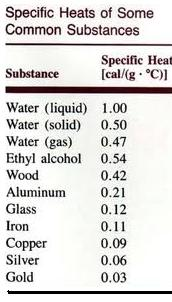• Have any questions?
• +91-9540-660064
• hahassignment@gmail.com

# Heat and Mass Transfer Assignment Help

### Introduction to heat and mass transfer:

Heat transfer occurs whenever there is homogeneous temperature distribution within materials or between material and its surroundings environments. There are three modes of heat transfer.

1. Conduction

2. Convection

### Conduction, Convection and Thermal Radiation

Conduction- Heat conduction in solids is by photons and free electrons and some transitional movements of atoms, in liquids is by phonons and free electrons and some transitional movements of atoms, while in gases is by random collision.

Convection- It possesses much larger heat transfer rate than heat conduction. There are two types of convection. Free convection and forced convection.Free convection is normally due to the temperature- density relationship. Forced convection is accelerated by external forces, such as using fan to blow the air in oven.

Thermal Radiation- thermal radiation is by electromagnetic propagation. There is no needed for thermal radiation, although the electromagnetic waves can be transferred through gases.

### Examples on Heat and Mass Transfer

In 1798, Count Rumford observed that the amount of heat is proportional to the amount of mechanical work done. If W is the amount of work done and Q is the quantity of heat produced, then

W~ Q

W= JQ, where J is the constant of proportionality known as Joule’s mechanical equivalent of heat or Joule’s constant

If Q=1, then J=W,

So, Joule’s mechanical equivalent of heat may be defined as amount of work required to be done to produce a unit quantity of heat.

If Q is measured in calorie and W in erg, then J= 4.186 * 10^ 7 erg/cal

If Q is measured in calorie and W in joule, then J= 4.186 joule /cal

In SI, both work and heat are two equivalent forms of energy. However the following distinction between work and heat must be clearly noted.

Work is the transfer of mechanical energy irrespective of temperature difference whereas heat is the transfer of thermal energy because of temperature difference only.

### Introduction to high specific heat:

Heat is a form of energy and it flows from a body at a higher temperature to a body at lower temperature. Therefore, when a hot body is placed in contact with a cold body, then the temperature of the hot body decreases and that of the cold body increases. This shows that a hot body loses heat and a cold body gains heat.The commonly used unit of heat is kilo calorie (k cal). A kilo calorie is the amount of heat required to raise the temperature of 1 kilogram of water through 1˚c, from 14.5˚c to 15.5˚c. This range of temperature is special in defining the unit quantity of heat energy, because water shows uniform and smooth expansion beyond 14˚c and slightly different quantities of heat are required for raising the temperature through 1˚c from different temperatures for 1kg of water.

### Units of Specific Heat

In CGS system the unit of heat is calorie and is defined as the quantity of heat required to raise the temperature of one gram of water through 1˚c.

1 kilo calorie=1000calories.

1 kilo calorie=4200 joules (J)

Or 1 calorie=4.2 joules (J)

Heat capacity

The heat required to raise the temperature through 1˚c depends upon the mass and also on the material of the substance. For example, if equal masses of water and copper are heated through 1˚c, then a large amount of heat has to be supplied to water.

### Heat Capacity

Different quantities of heat are required to change the temperature of same mass of different materials through the same range of temperatures. This shows that the different materials must have a property by virtue of which the same mass when heated through the same range of temperatures would absorb different quantities of heat.

This thermal property of the substance is called the specific heat capacity of the substance. It is also called the specific heat of the substance. It is defined as the quantity of heat energy required to raise the temperature of a unit mass of a substance through 1˚c.

Heat capacity=mass x specific heat capacity.

In case you face any problem or have any query please email us at :-info@homeworkassignmenthelp.com

### Submit us an Assignment:

Our tutors start working only after the payment is made, to ensure that we are doing work only for serious clients and also our solution meets the required standard.

### Getting homework help was never so easy you just need to follow following steps:

• Send us you Other Assignment or problem through email
• Specify the required format such as Word, Excel, Notepad, PDF
• Give us a deadline when you need the assignment completed along with the Time Zone.
(for example: EST, Australian GMT etc)
• Send documents related to your assignment which can help our tutors to provide a better work,
any example or format you want the solutions to be in.
• Our tutors will review the assignment sent by you and if all the required information is there we will
send you the price quoted by our tutor along with the time needed to solve the assignment
• You can pay us through paypal or credit card.
• After receiving the payment tutors start working on your assignment.
• Finally, we deliver the solutions and get a feedback from you regarding our work

In case you face any problem or have any query please email us at :- info@homeworkassignmenthelp.com